# Breadth First Search or BFS for a Graph

• Difficulty Level : Easy
• Last Updated : 27 Jun, 2022

Breadth-First Traversal (or Search) for a graph is similar to Breadth-First Traversal of a tree (See method 2 of this post). The only catch here is, that, unlike trees, graphs may contain cycles, so we may come to the same node again. To avoid processing a node more than once, we use a boolean visited array. For simplicity, it is assumed that all vertices are reachable from the starting vertex. BFS uses a queue data structure for traversal.

For example, in the following graph, we start traversal from vertex 2. When we come to vertex 0, we look for all adjacent vertices of it. 2 is also an adjacent vertex of 0. If we don’t mark visited vertices, then 2 will be processed again and it will become a non-terminating process.There can be multiple BFS traversals for a graph. Different BFS traversals for the above graph :
2, 3, 0, 1
2, 0, 3, 1

Recommended Practice

Following are the implementations of simple Breadth-First Traversal from a given source.
The implementation uses an adjacency list representation of graphs. STL‘s list container stores lists of adjacent nodes and the queue of nodes needed for BFS traversal.

## C++

 `// Program to print BFS traversal from a given``// source vertex. BFS(int s) traverses vertices``// reachable from s.``#include``using` `namespace` `std;` `// This class represents a directed graph using``// adjacency list representation``class` `Graph``{``    ``int` `V;    ``// No. of vertices` `    ``// Pointer to an array containing adjacency``    ``// lists``    ``vector> adj;  ``public``:``    ``Graph(``int` `V);  ``// Constructor` `    ``// function to add an edge to graph``    ``void` `addEdge(``int` `v, ``int` `w);` `    ``// prints BFS traversal from a given source s``    ``void` `BFS(``int` `s); ``};` `Graph::Graph(``int` `V)``{``    ``this``->V = V;``    ``adj.resize(V);``}` `void` `Graph::addEdge(``int` `v, ``int` `w)``{``    ``adj[v].push_back(w); ``// Add w to v’s list.``}` `void` `Graph::BFS(``int` `s)``{``    ``// Mark all the vertices as not visited``    ``vector<``bool``> visited;``    ``visited.resize(V,``false``);` `    ``// Create a queue for BFS``    ``list<``int``> queue;` `    ``// Mark the current node as visited and enqueue it``    ``visited[s] = ``true``;``    ``queue.push_back(s);` `    ``while``(!queue.empty())``    ``{``        ``// Dequeue a vertex from queue and print it``        ``s = queue.front();``        ``cout << s << ``" "``;``        ``queue.pop_front();` `        ``// Get all adjacent vertices of the dequeued``        ``// vertex s. If a adjacent has not been visited,``        ``// then mark it visited and enqueue it``        ``for` `(``auto` `adjecent: adj[s])``        ``{``            ``if` `(!visited[adjecent])``            ``{``                ``visited[adjecent] = ``true``;``                ``queue.push_back(adjecent);``            ``}``        ``}``    ``}``}` `// Driver program to test methods of graph class``int` `main()``{``    ``// Create a graph given in the above diagram``    ``Graph g(4);``    ``g.addEdge(0, 1);``    ``g.addEdge(0, 2);``    ``g.addEdge(1, 2);``    ``g.addEdge(2, 0);``    ``g.addEdge(2, 3);``    ``g.addEdge(3, 3);` `    ``cout << ``"Following is Breadth First Traversal "``         ``<< ``"(starting from vertex 2) \n"``;``    ``g.BFS(2);` `    ``return` `0;``}`

## Java

 `// Java program to print BFS traversal from a given source vertex.``// BFS(int s) traverses vertices reachable from s.``import` `java.io.*;``import` `java.util.*;` `// This class represents a directed graph using adjacency list``// representation``class` `Graph``{``    ``private` `int` `V;   ``// No. of vertices``    ``private` `LinkedList adj[]; ``//Adjacency Lists` `    ``// Constructor``    ``Graph(``int` `v)``    ``{``        ``V = v;``        ``adj = ``new` `LinkedList[v];``        ``for` `(``int` `i=``0``; i queue = ``new` `LinkedList();` `        ``// Mark the current node as visited and enqueue it``        ``visited[s]=``true``;``        ``queue.add(s);` `        ``while` `(queue.size() != ``0``)``        ``{``            ``// Dequeue a vertex from queue and print it``            ``s = queue.poll();``            ``System.out.print(s+``" "``);` `            ``// Get all adjacent vertices of the dequeued vertex s``            ``// If a adjacent has not been visited, then mark it``            ``// visited and enqueue it``            ``Iterator i = adj[s].listIterator();``            ``while` `(i.hasNext())``            ``{``                ``int` `n = i.next();``                ``if` `(!visited[n])``                ``{``                    ``visited[n] = ``true``;``                    ``queue.add(n);``                ``}``            ``}``        ``}``    ``}` `    ``// Driver method to``    ``public` `static` `void` `main(String args[])``    ``{``        ``Graph g = ``new` `Graph(``4``);` `        ``g.addEdge(``0``, ``1``);``        ``g.addEdge(``0``, ``2``);``        ``g.addEdge(``1``, ``2``);``        ``g.addEdge(``2``, ``0``);``        ``g.addEdge(``2``, ``3``);``        ``g.addEdge(``3``, ``3``);` `        ``System.out.println(``"Following is Breadth First Traversal "``+``                           ``"(starting from vertex 2)"``);` `        ``g.BFS(``2``);``    ``}``}``// This code is contributed by Aakash Hasija`

## Python3

 `# Python3 Program to print BFS traversal``# from a given source vertex. BFS(int s)``# traverses vertices reachable from s.``from` `collections ``import` `defaultdict` `# This class represents a directed graph``# using adjacency list representation``class` `Graph:` `    ``# Constructor``    ``def` `__init__(``self``):` `        ``# default dictionary to store graph``        ``self``.graph ``=` `defaultdict(``list``)` `    ``# function to add an edge to graph``    ``def` `addEdge(``self``,u,v):``        ``self``.graph[u].append(v)` `    ``# Function to print a BFS of graph``    ``def` `BFS(``self``, s):` `        ``# Mark all the vertices as not visited``        ``visited ``=` `[``False``] ``*` `(``max``(``self``.graph) ``+` `1``)` `        ``# Create a queue for BFS``        ``queue ``=` `[]` `        ``# Mark the source node as``        ``# visited and enqueue it``        ``queue.append(s)``        ``visited[s] ``=` `True` `        ``while` `queue:` `            ``# Dequeue a vertex from``            ``# queue and print it``            ``s ``=` `queue.pop(``0``)``            ``print` `(s, end ``=` `" "``)` `            ``# Get all adjacent vertices of the``            ``# dequeued vertex s. If a adjacent``            ``# has not been visited, then mark it``            ``# visited and enqueue it``            ``for` `i ``in` `self``.graph[s]:``                ``if` `visited[i] ``=``=` `False``:``                    ``queue.append(i)``                    ``visited[i] ``=` `True` `# Driver code` `# Create a graph given in``# the above diagram``g ``=` `Graph()``g.addEdge(``0``, ``1``)``g.addEdge(``0``, ``2``)``g.addEdge(``1``, ``2``)``g.addEdge(``2``, ``0``)``g.addEdge(``2``, ``3``)``g.addEdge(``3``, ``3``)` `print` `(``"Following is Breadth First Traversal"``                  ``" (starting from vertex 2)"``)``g.BFS(``2``)` `# This code is contributed by Neelam Yadav`

## C#

 `// C# program to print BFS traversal``// from a given source vertex.``// BFS(int s) traverses vertices``// reachable from s.``using` `System;``using` `System.Collections.Generic;``using` `System.Linq;``using` `System.Text;` `// This class represents a directed``// graph using adjacency list``// representation``class` `Graph{``    ` `// No. of vertices    ``private` `int` `_V;` `//Adjacency Lists``LinkedList<``int``>[] _adj;` `public` `Graph(``int` `V)``{``    ``_adj = ``new` `LinkedList<``int``>[V];``    ``for``(``int` `i = 0; i < _adj.Length; i++)``    ``{``        ``_adj[i] = ``new` `LinkedList<``int``>();``    ``}``    ``_V = V;``}` `// Function to add an edge into the graph``public` `void` `AddEdge(``int` `v, ``int` `w)``{        ``    ``_adj[v].AddLast(w);` `}` `// Prints BFS traversal from a given source s``public` `void` `BFS(``int` `s)``{``    ` `    ``// Mark all the vertices as not``    ``// visited(By default set as false)``    ``bool``[] visited = ``new` `bool``[_V];``    ``for``(``int` `i = 0; i < _V; i++)``        ``visited[i] = ``false``;``    ` `    ``// Create a queue for BFS``    ``LinkedList<``int``> queue = ``new` `LinkedList<``int``>();``    ` `    ``// Mark the current node as``    ``// visited and enqueue it``    ``visited[s] = ``true``;``    ``queue.AddLast(s);        ` `    ``while``(queue.Any())``    ``{``        ` `        ``// Dequeue a vertex from queue``        ``// and print it``        ``s = queue.First();``        ``Console.Write(s + ``" "` `);``        ``queue.RemoveFirst();``        ` `        ``// Get all adjacent vertices of the``        ``// dequeued vertex s. If a adjacent``        ``// has not been visited, then mark it``        ``// visited and enqueue it``        ``LinkedList<``int``> list = _adj[s];` `        ``foreach` `(``var` `val ``in` `list)            ``        ``{``            ``if` `(!visited[val])``            ``{``                ``visited[val] = ``true``;``                ``queue.AddLast(val);``            ``}``        ``}``    ``}``}` `// Driver code``static` `void` `Main(``string``[] args)``{``    ``Graph g = ``new` `Graph(4);``    ` `    ``g.AddEdge(0, 1);``    ``g.AddEdge(0, 2);``    ``g.AddEdge(1, 2);``    ``g.AddEdge(2, 0);``    ``g.AddEdge(2, 3);``    ``g.AddEdge(3, 3);``    ` `    ``Console.Write(``"Following is Breadth First "` `+``                  ``"Traversal(starting from "` `+``                  ``"vertex 2)\n"``);``    ``g.BFS(2);``}``}` `// This code is contributed by anv89`

Output

```Following is Breadth First Traversal (starting from vertex 2)
2 0 3 1 ```

Time Complexity: O(V+E), where V is the number of nodes and E is the number of edges.
Auxiliary Space: O(V)

Illustration :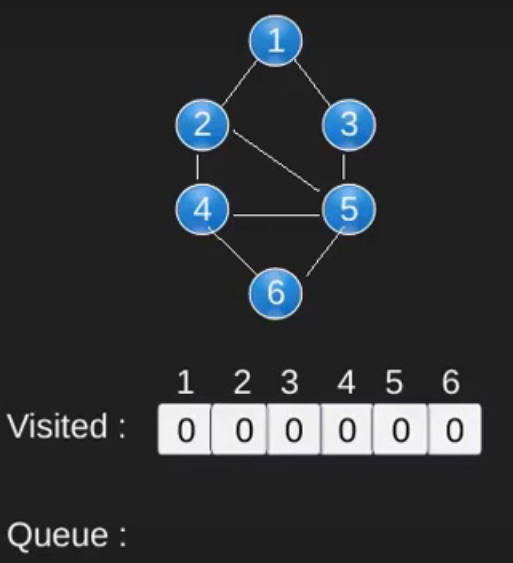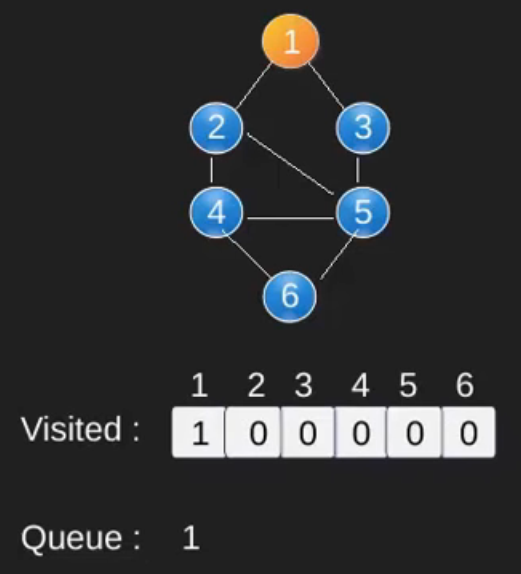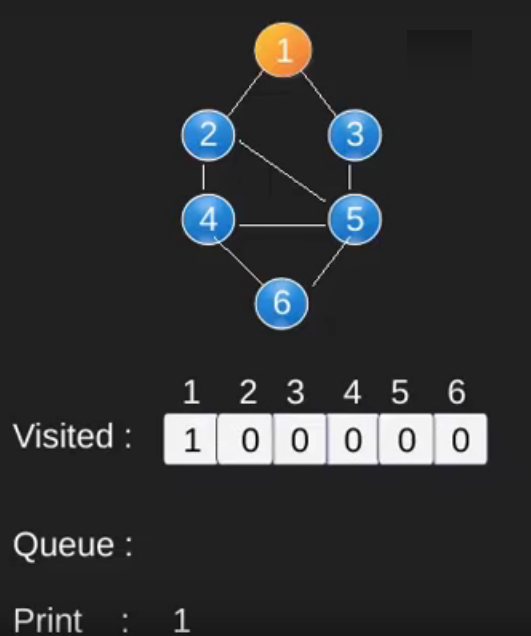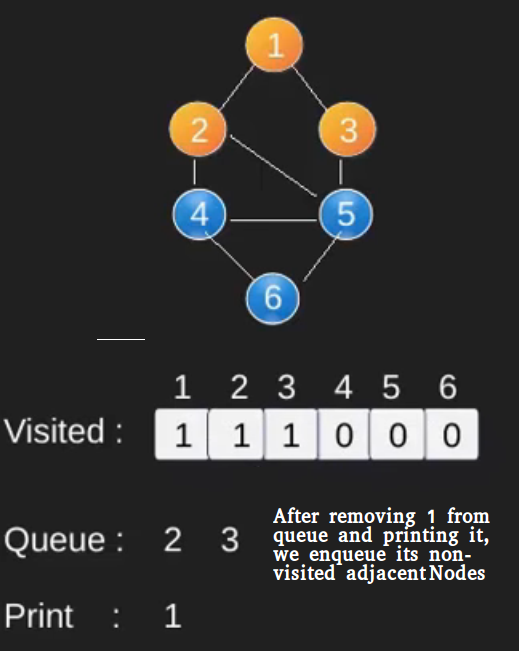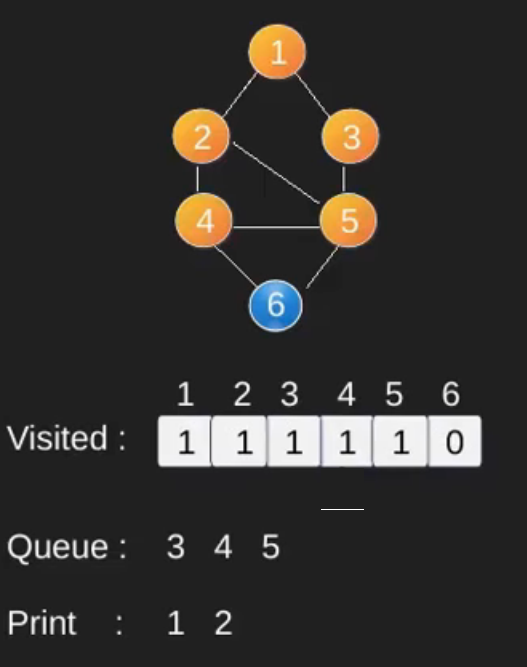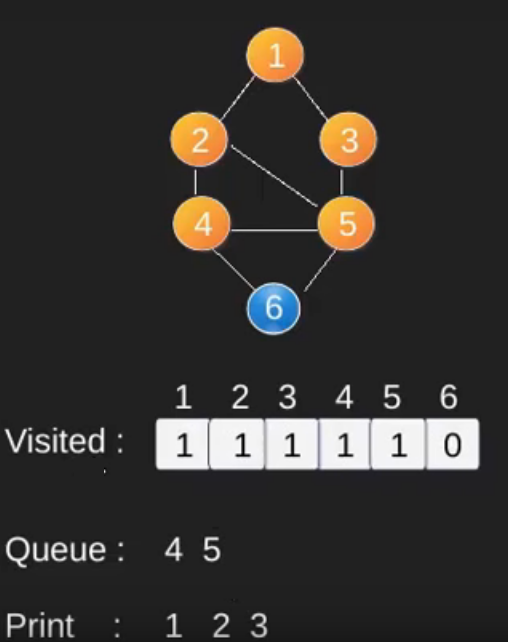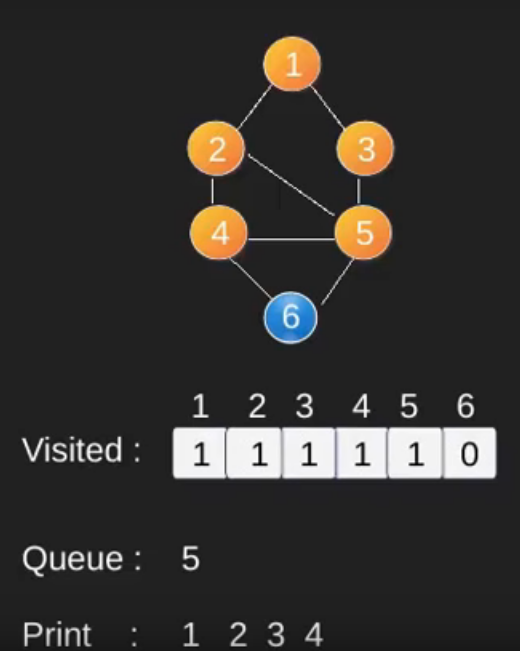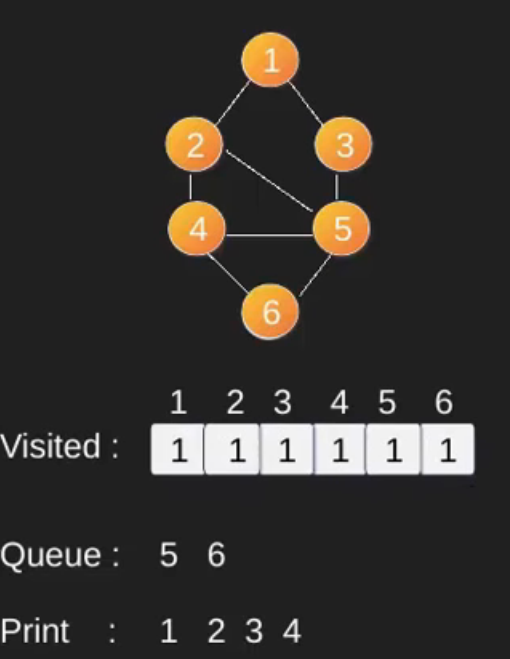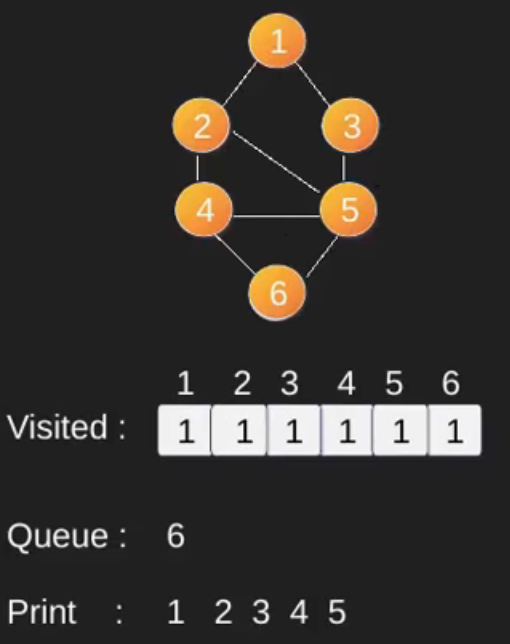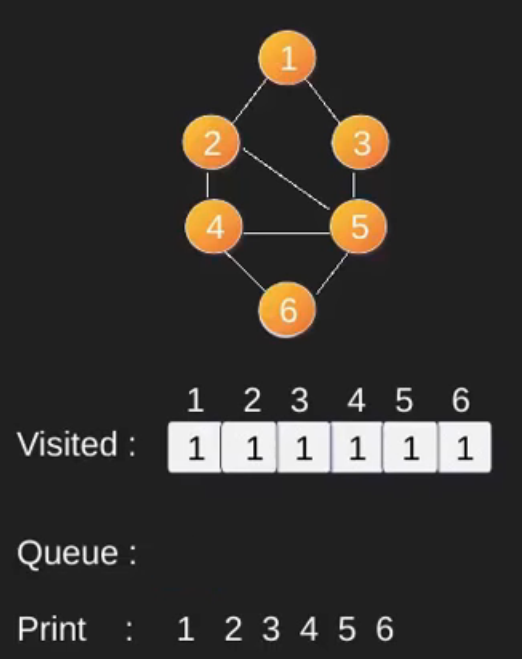Note that the above code traverses only the vertices reachable from a given source vertex. The vertices may not be reachable from a given vertex (for example Disconnected graph).

To print all the vertices, we can modify the BFS function to do traversal starting from all nodes one by one (Like the DFS modified version).

The C++ code for BFS traversal for the entire graph (valid for directed as well as undirected graphs) with possible multiple disconnected components is as follows:

## C++

 `/*******************************************************`` ``* Generic Function for BFS traversal of a Graph`` ``* (valid for directed as well as undirected graphs`` ``*  which can have multiple disconnected commponents)`` ``*`` ``********** Inputs *************************************`` ``* V - represents number of vertices in the Graph`` ``* adj[] - represents adjacency list for the Graph`` ``*`` ``********** Output *************************************`` ``* bfs_traversal - a vector containing bfs traversal`` ``*                 for entire graph`` ``*******************************************************/` `vector<``int``> bfsOfGraph(``int` `V, vector<``int``> adj[])``{``    ``vector<``int``> bfs_traversal;``    ``vector<``bool``> vis(V, ``false``);``    ``for` `(``int` `i = 0; i < V; ++i) {``        ``if` `(!vis[i]) {``            ``queue<``int``> q;``            ``vis[i] = ``true``;``            ``q.push(i);``            ``while` `(!q.empty()) {``                ``int` `g_node = q.front();``                ``q.pop();``                ``bfs_traversal.push_back(g_node);``                ``for` `(``auto` `it : adj[g_node]) {``                    ``if` `(!vis[it]) {``                        ``vis[it] = ``true``;``                        ``q.push(it);``                    ``}``                ``}``            ``}``        ``}``    ``}``    ``return` `bfs_traversal;``}`

You may like to see below also :